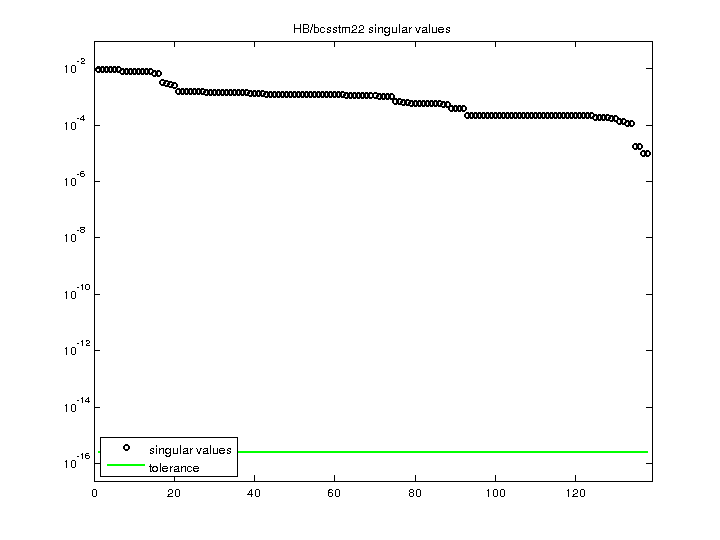Matrix: HB/bcsstm22

Description: SYMMETRIC MASS MATRIX - TEXTILE LOOM FRAME(undirected graph drawing)• Matrix group: HB
• download as a MATLAB mat-file, file size: 1 KB. Use UFget(72) or UFget('HB/bcsstm22') in MATLAB.

 Matrix properties number of rows 138 number of columns 138 nonzeros 138 structural full rank? yes structural rank 138 # of blocks from dmperm 138 # strongly connected comp. 138 explicit zero entries 0 nonzero pattern symmetry symmetric numeric value symmetry symmetric type real structure symmetric Cholesky candidate? yes positive definite? yes

 author J. Lewis editor I. Duff, R. Grimes, J. Lewis date 1984 kind structural problem 2D/3D problem? yes

 Ordering statistics: result nnz(chol(P*(A+A'+s*I)*P')) with AMD 138 Cholesky flop count 1.4e+02 nnz(L+U), no partial pivoting, with AMD 138 nnz(V) for QR, upper bound nnz(L) for LU, with COLAMD 138 nnz(R) for QR, upper bound nnz(U) for LU, with COLAMD 138

 SVD-based statistics: norm(A) 0.00973767 min(svd(A)) 1.0345e-05 cond(A) 941.295 rank(A) 138 sprank(A)-rank(A) 0 null space dimension 0 full numerical rank? yes

 singular values (MAT file): click here SVD method used: s = svd (full (A)) ; status: ok